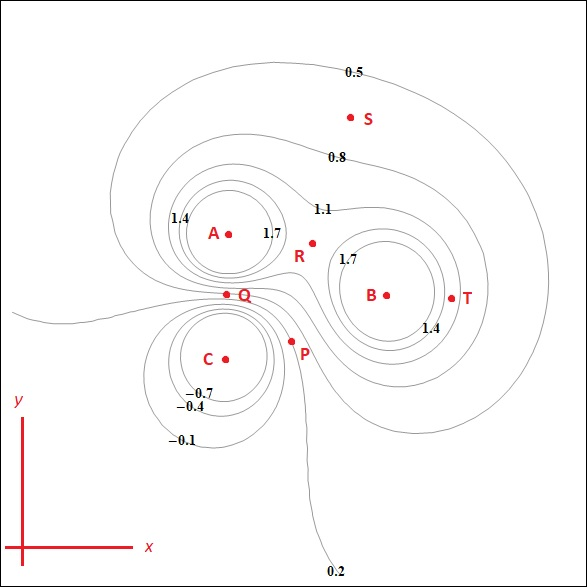# 0.5S0.81,4A.1.7R17QВеT14PC-0.7-0.40.1X0.2

Question
13 views
 The figure on the right shows plot of the equipotentials for an arrangement of charges. The numbers represent the potentials (in volts) of each of the equipotential lines. Equipotential lines are shown for potentials beginning at -0.7 V and increasing by 0.3 V up to 1.7 V. There could be equipotential lines for potentials less than -0.7 or greater than 1.7, but these are not shown for clarity. 4. At which point or points is the electric field zero?P Q R S T None5. At which point or points would the electric field be weakest but non-zero?P Q R S T None6. At which point or points would the electric field point have +x and +y components (i.e., the electric field points up and to the right)?P Q R S T Nonehelp_outlineImage Transcriptionclose0.5 S 0.8 1,4 A. 1.7 R 17 Q Ве T 14 P C -0.7 -0.4 0.1 X 0.2 fullscreen
check_circle

Step 1

Sub-Part (4)

Electric Field Lines can be defined as a curve which shows direction of electric field, when we draw tangent at its point.

Step 2

R has zero electric field as the field arriving from both positive chargers are coming in opposite directions and thus would be cancelling out each other.

Step 3

Sub-Part (5)

P point will have the least but non zero electric field because the magnitude of point P is 0.2 where as for other points are, Q ha...

### Want to see the full answer?

See Solution

#### Want to see this answer and more?

Solutions are written by subject experts who are available 24/7. Questions are typically answered within 1 hour.*

See Solution
*Response times may vary by subject and question.
Tagged in

### Physics